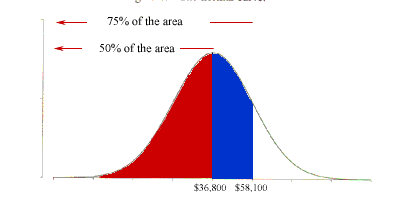Quandaries and Queries My name: Gary I am a student. The level of the question: College. I had several questions regarding a percentile grid, and I just wanted to make sure I answered them correctly. Table 1 Selected percentiles for family income in the US in 1992 1 \$1,300 10 \$10,200 25 \$20,100 50 \$36,800 75 \$58,100 90 \$85,000 99 \$151,800 Q. The percentage of families in Table 1 with incomes below \$58,100 was about? I put 50%. Q. About 10% of the families in Table 1 had incomes below? I put \$10,200. Q. The percentage of families in Table 1 with incomes between \$10,200 and \$85,000 was about? I put 85%. Q. The percentage of families in Table 1 with incomes between \$36,800 and \$58,000 was about? I put 25%. Could you please verify for me that my answers are correct? Thanks, Gary Hi Gary, I drew a diagram to show the fiftieth percentile and the seventy-fifth percentile.The fiftieth percentile is \$36,800 so 50% of the area under the curve is to the left of \$36,800 (the region shaded red). The seventy-fifth percentile is \$58,100 so 75% of the area under the curve is to the left of \$58,100. Hence the region shaded blue is 25% of the area. Hence the answer to the first question is 75%. Penny Go to Math Central